# Chapter 2 EXAMPLES Example 1 Consider a river

• Slides: 9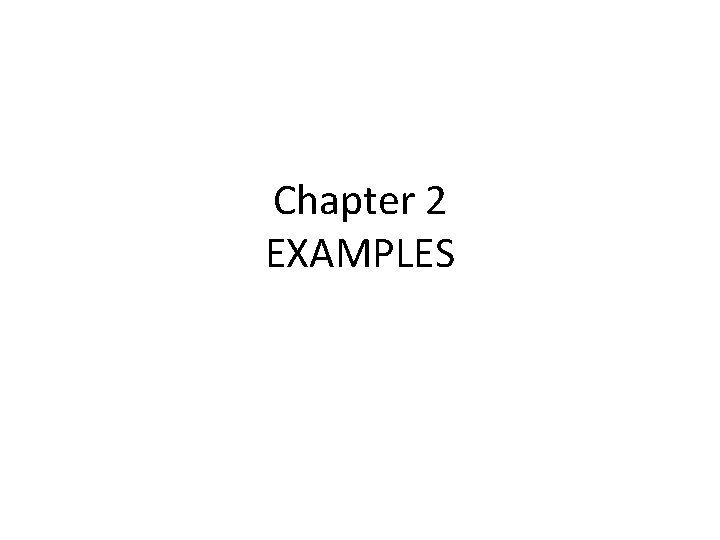Chapter 2 EXAMPLES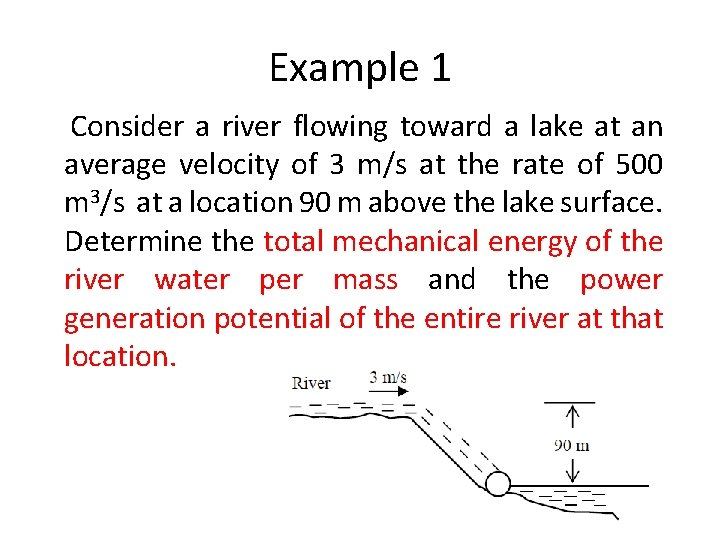Example 1 Consider a river flowing toward a lake at an average velocity of 3 m/s at the rate of 500 m 3/s at a location 90 m above the lake surface. Determine the total mechanical energy of the river water per mass and the power generation potential of the entire river at that location.Example 2 Consider a fan located in a 1 m x 1 m square duct. Velocity at various points at the outlet are measured, and the average flow velocity is determined to be 7 m/s. Taking the air density to 1. 2 kg/m 3, estimate the minimum electric power consumption of the fan motor.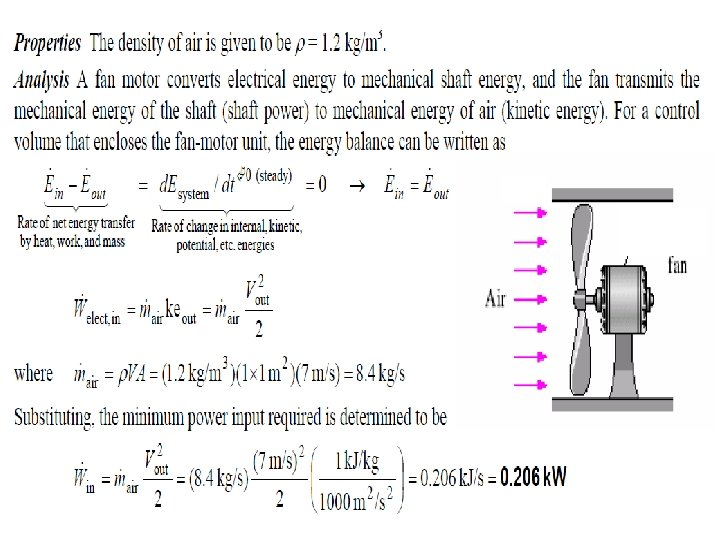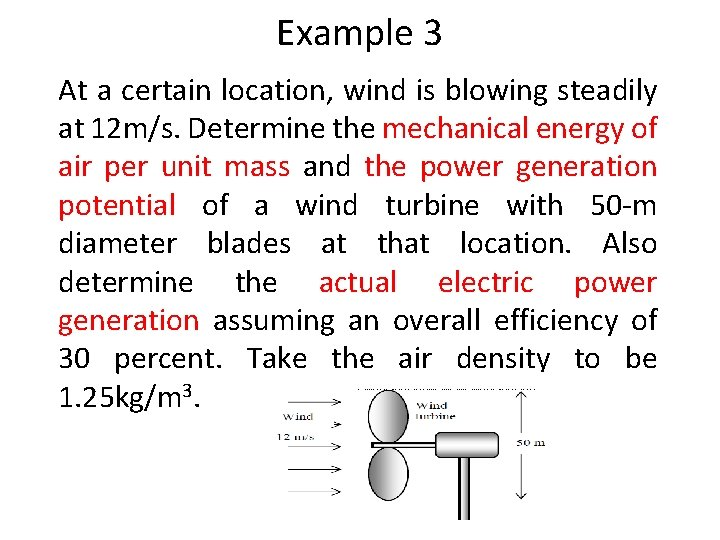Example 3 At a certain location, wind is blowing steadily at 12 m/s. Determine the mechanical energy of air per unit mass and the power generation potential of a wind turbine with 50 -m diameter blades at that location. Also determine the actual electric power generation assuming an overall efficiency of 30 percent. Take the air density to be 1. 25 kg/m 3.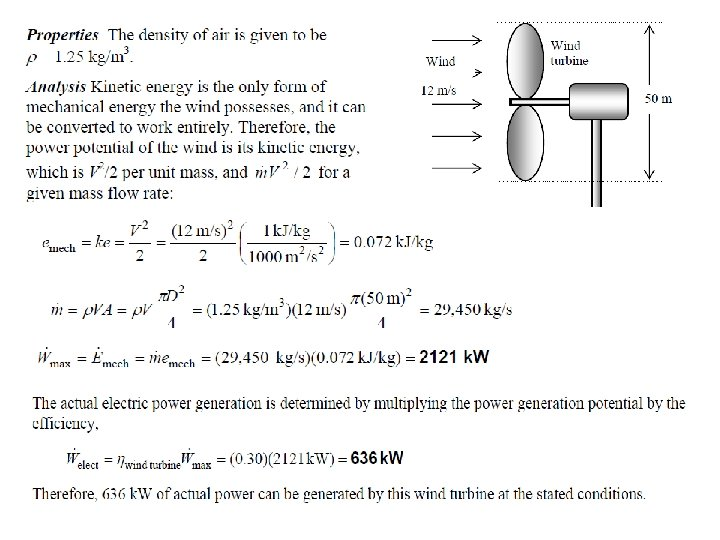Example 4 Electric power is to be generated by installing a hydraulic turbine-generator at a site 70 m below the free surface of a large water reservoir that can supply water at a rate of 1500 kg/s steadily. If the mechanical power output of the turbine is 800 k. W and the electric power generation is 750 k. W, determine the turbine efficiency of this plant. Neglect losses in the pipes.